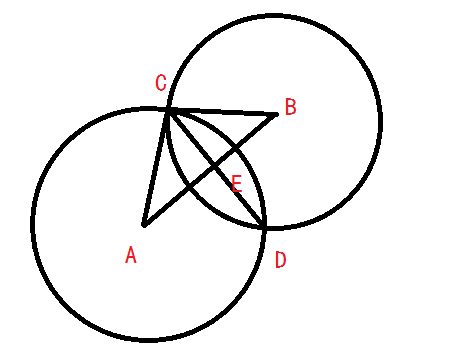# 基于rssi的三点定位算法

首先是将rssi信号转换为距离：

d=10^((ABS(RSSI)-A)/(10*n))

其中d为距离，单位是m。

RSSI为rssi信号强度，为负数。

A为距离探测设备1m时的rssi值的绝对值，最佳范围在45-49之间。

n为环境衰减因子，需要测试矫正，最佳范围在3.25-4.5之间。

在获取未知点到三个点的距离后，剩下的就是求解未知点的坐标。我们都知道两个圆会相交于一个或者两个点（如果相交），那么三个圆如果相交的话，必然会交于一个点（三个探测设备在一条直线上的情况下有可能相交于两个点，这里不考虑），所以我们要求解的未知点便是以三个已知点为圆心，以他们与未知点之间的距离为半径画出的三个圆的交点。那么这个问题就转化为了求三个已知圆的交点，然后如果根据圆的方程：

(x1 – x)^2 + (y1-y)^2 = r1^2

(x2 – x)^2 + (y2-y)^2= r2^2

(x3 – x)^2 + (y3-y)^2= r3^2

(x1 – x2)^2 + (y1-y2)^2= (r1+r2+d)^2

x = x1+ (x2 - x1)*(r1/(r1 + r2));

y = y1 + (y2- y1)*(r1/(r1 + r2));

二、没有任意两个圆相切，那么就先用两个圆求解两个交点，如下图:其中A,B是两个圆心，坐标分别为（xaya）和（xbybs），C,D是两个圆的焦点，E为AB与CD的交点。

其中

AB^2 = (xa – xb)^2 +(ya-yb)^2

其中

AC^2 =AE^2 + CE^2               ……………………1

BC^2 = BE^2+ CE^2                                ……………………2

AC = ra

BC= rb

AE + BE = AB = (xa – xb)^2+ (ya – yb)^2

等式2转换为

BC^2 = (AB - AE)^2 + CE^2

BC^2 = AB^2 + AE^2 – 2*AB*AE + CE^2 ……………………3

BC^2 - AC^2 = AB^2 – 2*AB*AE

AE = (rb ^2 - ra ^2 -AB^2)/( – 2*AB)

CE^2 = AC^2 – AE^2

xE = xa + ( (xb- xa)*AE )/AB

yE = ya + ( (yb- ya)*AE )/AB

kAB = (yb - ya)/(xb - xa)

kCD = (-1)/kAB //这里要注意kAB为0的情况

∠CDX = atan(kCD)

xc = xE + CE*cos(∠CDXs)

yc = yE + CE*sin (∠CDXs)

xd = xE - CE*cos(∠CDXs)

yd = yE - CE*sin (∠CDXs) //这里也要注意sin (∠CDXs)和cos(∠CDXs)为nan的情况

09-0303-29
04-01
03-22
08-012万+
04-30
01-17
03-071万+
05-283336
05-09
12-301147
09-05
05-14
12-21162
01-298352
12-021万+
07-077586
12-30663
©️2020 CSDN 皮肤主题: Age of Ai 设计师:meimeiellie